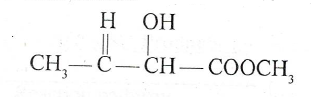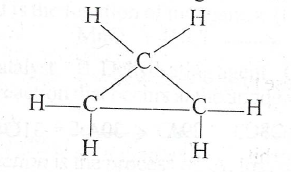# (a)(i) Define the term functional group. (ii) Name the functional groups present in the following compound: (b) Consider the following structure of an organ...

Question 1

(a)(i) Define the term functional group.

(ii) Name the functional groups present in the following compound:(b) Consider the following structure of an organic compound, Q.(1) Name compound Q.

(ii) Write the balanced equation for the complete combustion of compound Q.

(iii) What type of reaction will compound Q undergo with chlorine?

(vi) Draw the structure of-the alkene that is an isomer of compound Q.

(c) A chemistry student was provided with four samples of organic compounds, A, B, C, and D. Samples A and B each decolourized bromine in tetrachloromethane but only sample B reacted with a solution of ammoniacal silver trioxonitrate (V) to give a white precipitate. Sample D reacted with sodium trioxocarbonate (IV) to liberate carbon (IV) oxide. When heat was applied to the mixture of samples C and D in a test tube and drops of concentrated tetraoxosulphate (VI) acid added, a product with a fruity odour was formed.

(i) Name the family of organic compounds to which samples A, B, C, and D belong.

(ii) State why samples A and B reacted with bromine in tetrachloromethane.

(iii) State the reason why sample B gave a white precipitate with the solution of ammoniacal silver trioxocarbonate (V).

(iv) Give the name o f the reactions between' samples C and D.

(v) State the two roles of the concentrated tetraoxosulphate (VI) acid in the reaction in (a)(iv).

(d) (i) Describe briefly the production of biogas using a biogas generator.

(ii) State two uses of biogas.